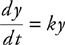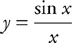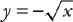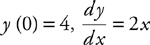# AP Calculus BC Practice Test 40

### Test Information7 questions21 minutes

Calculator Allowed

1. The amount of a certain bacteria y in a Petri dish grows according to the equation, where k is a constant and t is measured in hours. If the amount of bacteria triples in 10 hours, then k

2. The area of the region enclosed by the graphs of y = cos x + 1 and y = 2 + 2x - x2 is approximately

3. How many points of inflection does the graph ofhave on the interval (-π, π)?

4. Given f (x) = x2ex, what is an approximate value of f (1.1), if you use a tangent line to the graph of f at x = 1?

5. The area of the region bounded by y = 3x2 - kx + 1 and the x-axis, the lines x = 1 and x = 2 is approximately -5.5. Find the value of k.

6. At what value(s) of x do the graphs of y = x2 andhave perpendicular tangent lines?

7. Using Euler’s Method, what is the approximate value of y (1) if, and a step size of 0.5 starting at x = 0?